lvy>試卷(2022/08/16)

# 103 年 - 103 國立中山大學_碩士班招生考試_電機系(甲、丁、戊、己組)：工程數學甲#110234

【非選題】
1.

1. (15%) Evaluate the following integral【非選題】
2.

2. (15%) Deine the Fourier transform ofa signal f(t) as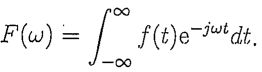【題組】

(2) (7%6) Compute the quantity A given belowwhere the Fourier transform of f1(t) is given in the following figure (a).【非選題】
3.【題組】

(b) (8%) Compute the quantity B given belowwhere the Fourier transform of f2(t) is given in the following figure (b).【非選題】
4.

3.(11%)下面的問题共有二個子題,(1)子題要清楚地寫山證明,(b)子題只要簡短扼要地回答 提問即可。 Let A be any matrix in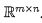. Then, since rank
(A) = dim(R
(A)), the dimension of range of A, and R
(A) = R(AAT), we have the result rank
(A) = rank(AAT). Therefore, when replacing A by its QR factorization, we get rank
(A) = rank(QRRTQT).

【題組】

(a) (6%) Please continue the argument to derive the result rank
(A) = rank(R).
(接下來前段是背景知識介紹,之後才是提問)Insthq given, instead of using the elementary row operations (i.e. the Gauss eliminations) to manipulate the equation, we may also apply the QR factorization to the equation to get QRx = b, which implies further QT QRx = QTb. Since QTQ = In, it gives Rx = QTb. Thus, according to the result of (a), when all columns of A are linearly independent, the square matrix R is nonsingular and so the solution x =b is obtained. It seems that we may summarize the above argument as the following statement:

Given, where all columns of A are assumed linearly independent, then solution to the equation Ax = 6 can always be computed from x =, where Q and R are matrices obtained from the QR factorization of A.

However, the simple exampleshows that the summary is incorrect because, according to the summary, the solution is x ==0 and obviously it does not satisfy the original equation.

【非選題】
5.【題組】(b) (5%) What is the error (or are the errors) in the argument right before the summary to make it incorrect?

【非選題】
6.

4.(14%)下面的問題共有二個子題,只要簡短扼要地回答提問即可,不須寫出答案背後的推 導。
Let V be a vector space with dim(V) = n and an ordered basis E := [x1, ..,xn].Let F := [y1,... , Yn] be the ordered orthonormal basis generated from basis E by applying the Gran- Schmidt Orthogonalization Process. For any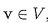, let [v]Eand [v]F denote the coordinate vectors of v with respect to bases E and F, respectively. Let T denote the transition matrix from basis E to basis F. Let L :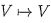, ie. L is a linear operator mapping V into itself, and suppose that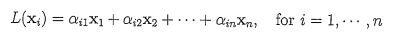Let's denote the matrix representation of L with respect to basis E by A.

【題組】(a) (6%) Write an equation to indicate the relationship between the two coordinate vectors [L(v)]E and [v]F.

【非選題】
7.【題組】(b) (8%) Obviously, matrix T' relates to the two bases E and F. What conclusions about vectors in basis E and/or in basis F can be drawn if the matrix Tis known to be diagonal?

【非選題】
8.

5. (25%) Consider the following system of differential equations: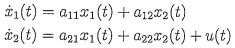where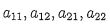are constant coefficients.

【題組】

(a) (10%) Let. Suppose4 0 and u ≡ 0. Find the solution of x1 and x2 for the initial conditions x1(0) =.

【非選題】
9.【題組】

(b) (4%)SupposeFind the range of k: such that the solution of x1 and x2 converges to zero for any initial condition.

【非選題】
10.【題組】

(c) (5%) SupposeFind the range of k such that the solution of at and ta exhibits oscillatory behavior for any nonzero initial condition.

【非選題】
11.【題組】

(d) (6%) For the values, calculate the steady-state response of.

【非選題】
12.
6.(15%) Let F(c, y2Z) = (y +y2z)i +(2-z+2xyz)j + (-y +xy2)k

【題組】(a) (3%) Verify that F' is conservative.

【非選題】
13.【題組】(b) (10%) Find a potential function f(x, y, z) for F(c, y, z).

【非選題】
14.【題組】

(c) (2%) Find, where C is the straight line going from the points (2,2,1) to the point (1,-1,2).

【非選題】
15.

7. (5%) Evaluate the following integral